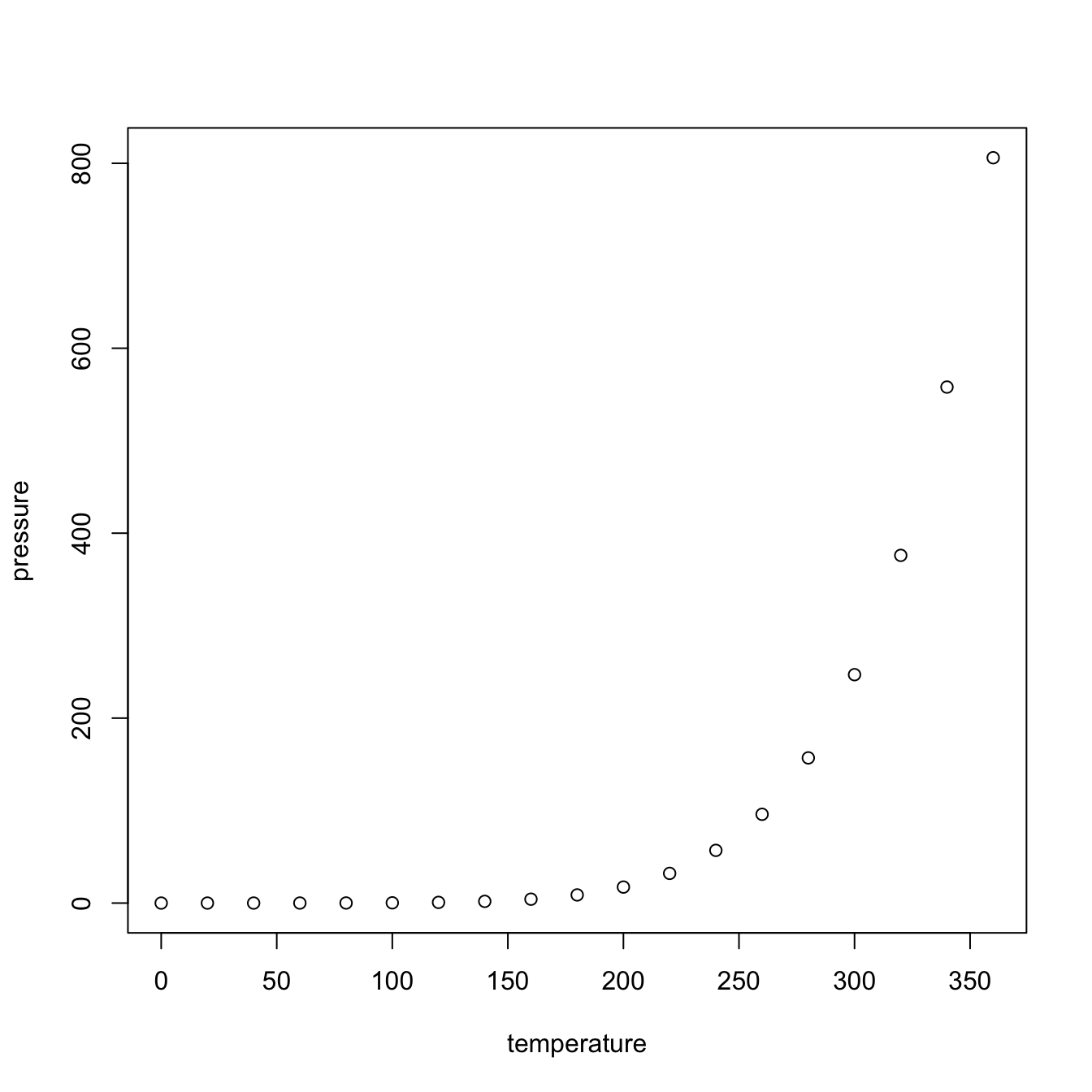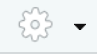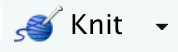When using R for data analysis and visualization, one may discover that the default parameter settings produce too small labels and titles for including in our articles and report. This tutorial provides a few tricks and guidelines for producing visualizations that are better readable. The tutorial is based on producing plots using R’s basic plot functions (see here for more info).

We will use as working code R Markdown’s example plot, which looks like this if we use the default values and styling and set the output size to 300 x 300 px:

``plot(pressure)``Note that the axes labels and tick labels are rather small. There are (at least) two different aspects that may change the size of the labels, namely the size of the plot panel and the parameter `cex`. We will discuss both of these below, after illustrating how to save an R plot to file.

All visualizations in this output file are set to a height of 300px to facilitate comparison with the original plot.

## Changing the size of the plot

By default, the plot panel is set to 7 x 7 inches. When we would reduce the size of the plot panel but keep the font sizes constant, the labels automatically will appear larger in the resulting plot.

However, when working in R Studio we cannot change the size of the plot when we send the plot to the plot pane. Instead, we may want to have the plots appearing just after the code blocks in our R Markdown /R Notebook reports, or send the plot to a separate plot device. Both options are explained below.

#### … when using R Markdown / R Notebook

Open your R Markdown or R Notebook file in R Studio and click on the wheelright to the knit symbol, and select the option “Chunk output inline” from the drop-down menu. With this option selected instead of “Chunk output in Console” the plots will appear below the code blocks in the report rather than in the R Studio plot pane.

Alternatively – for example when not working in R Studio – you could add the following lines to the header:

``````editor_options:
chunk_output_type: console``````

Now we have made sure that the plots will appear inline, following the code blocks, we can change the size of the plots in the settings of our code block. I prefer a default plot panel of 4 x 4 inches (instead of 7 x 7 inches). Add `fig.width=4, fig.height=4` to the settings of the code block:

`` ```{r myplot, fig.width=4, fig.height=4}``

This result in the following plot (with `out.width=“300px” added to ensure the same size as the original plot):

``plot(pressure)``